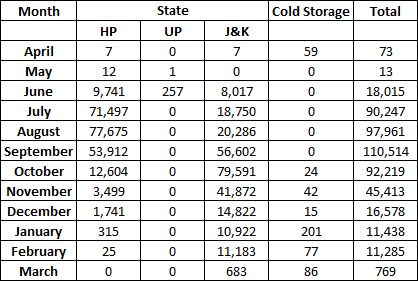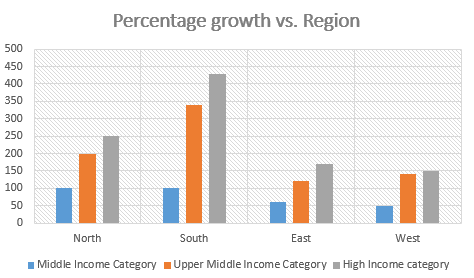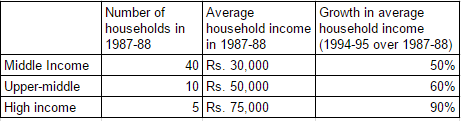# CAT 1998

Instructions

The following table gives the quantities of apples (in tonnes) arriving at New Delhi market from various states in a particular year. The month in which demand was more than supply, the additional demand was met by the stock from cold storage.Question 1

What was the maximum percentage of apples supplied by any state in any of the months?

Question 2

Which state supplied the maximum number of apples?

Question 3

Which state supplied the highest percentage of apples from the total apples supplied?

Question 4

In which of the following periods was the supply greater than or equal to the demand?

Question 5

If the yield per tree was 40 kg, then from how many trees were the apples supplied to New Delhi (in millions) during the year?

Question 6

Using the data in the earlier question, if there were 250 trees per hectare, then how many hectares of land was used?

Instructions

Answer the questions based on the following information. The following bar chart gives the growth percentage in the number of households in the middle, upper-middle and high-income categories in the four regions for the period between 1987-88 and 1994-95.(Number of households in thousands)

Question 7

Which region showed the highest percentage growth in number of households in all the income categories for the period?

Question 8

What was the total household income in northern region for upper-middle class?

Question 9

What is the percentage increase in total number of households for the northern region (upper-middle class) over the given period?

Question 10

What was the average income of the high-income group in 1987-88?

OR